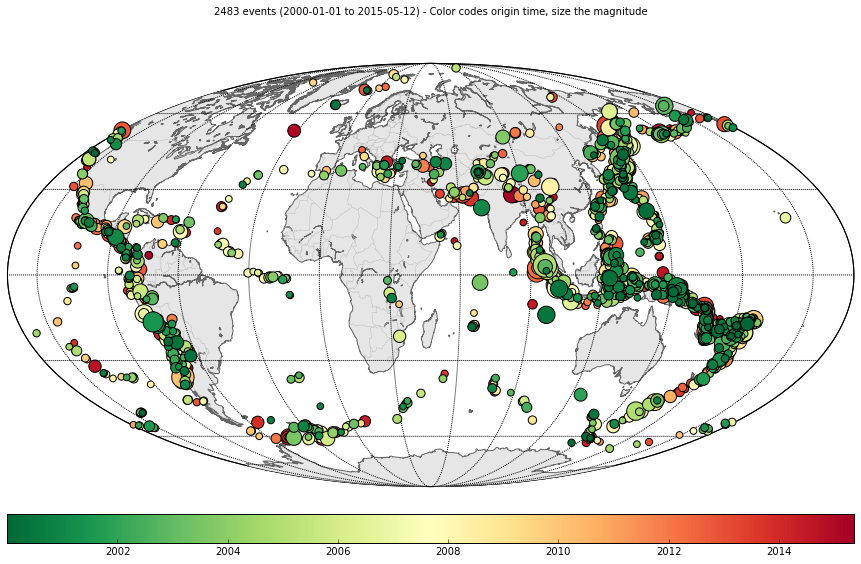This is a very short attempt at looking at a Python conversion of this MATLAB Central post. I won't be converting the text and explanations; just trying out the examples.

In :
%matplotlib inline

import numpy as np
import matplotlib.pyplot as plt
plt.rcParams['figure.figsize'] = 12, 8

Examples¶

The ObsPy package contains several methods that can used to retrieve station metadata, time series, or event information from FDSN servers. They are the get_stations, get_waveforms and get_events methods of the obspy.fdsn.Client class. Below are some examples of how each of these components can be used for common data retrieval tasks.

In :
from obspy import UTCDateTime
from obspy.fdsn import Client

client = Client('IRIS', debug=True)
Base URL: http://service.iris.edu
Request Headers: {'User-Agent': 'ObsPy 0.10.2 (Linux-3.19.7-200.fc21.x86_64-x86_64-with-fedora-21-Twenty_One, Python 3.4.1)'}
Discovered dataselect service
Discovered event service
Discovered station service
Storing discovered services in cache.

Example 1¶

Plot time series for the 2011 Tohoku-Oki earthquake. The following example uses get_waveforms to retrieve time series data for global GSN stations to plot 1 hour of data (bandpass filtered from 1 - 5 Hz) following the Tohoku-Oni earthquake of March 2011.

In :
start = UTCDateTime('2011-03-11T15:10:00')
end = UTCDateTime('2011-03-11T16:10:00')

tohoku_tr = client.get_waveforms(network='_GSN', station='MAJO,WMQ,KAPI,CMB',
location='00', channel='BHZ',
starttime=start, endtime=end)
In :
tohoku_tr.plot(equal_scale=False)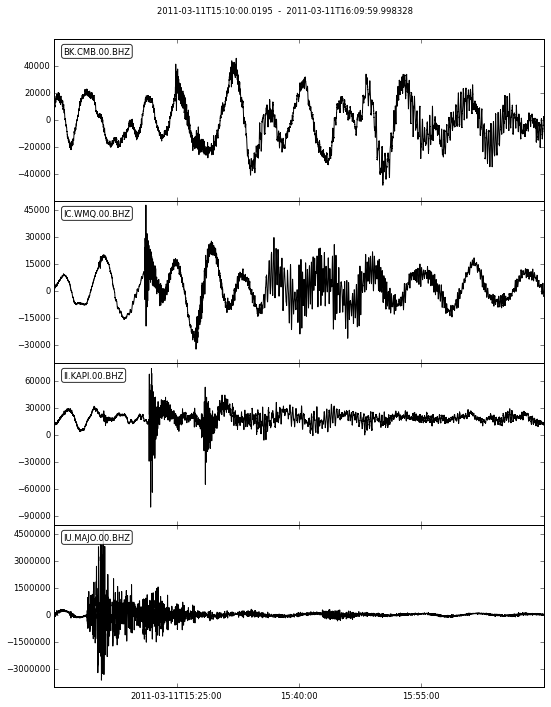set filter parameters

In :
bandfilt_freq1 = 1
bandfilt_freq2 = 5
bandfilt_order = 4
In :
tohoku_filt = tohoku_tr.filter(type='bandstop', freqmin=bandfilt_freq1, freqmax=bandfilt_freq2, corners=bandfilt_order)
In :
tohoku_filt.label = 'Tohoku-Oni Earthquake, 11-March-2011'
tohoku_filt.plot(equal_scale=False)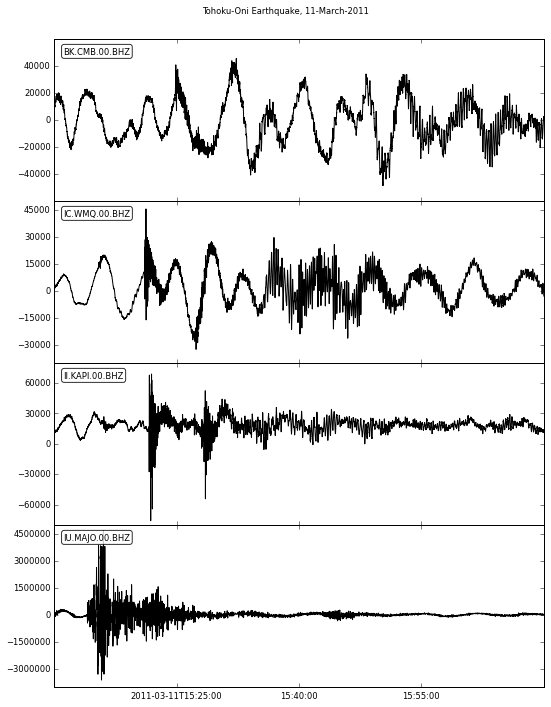Example 2¶

Plot a map of Transportable Array (TA) network stations and recent earthquakes in Oklahoma. This example uses get_events and get_stations to retrieve hypocenter data and station metadata, respectively, to make a simple plot.

In :
minlat = 35
maxlat = 37.5

minlon = -100
maxlon = -95

starttime = UTCDateTime('2012-01-01T00:00:00')
endtime = UTCDateTime('2015-02-01T00:00:00')

minmag = 0.0
maxmag = 4.0

ok_ev = client.get_events(minlatitude=minlat, maxlatitude=maxlat,
minlongitude=minlon, maxlongitude=maxlon,
minmagnitude=minmag, maxmagnitude=maxmag,
starttime=starttime, endtime=endtime)

ok_sta = client.get_stations(network='TA', channel='BH?',
minlatitude=minlat, maxlatitude=maxlat,
minlongitude=minlon, maxlongitude=maxlon)

title = 'M<%d -- %s to %s -- %d total events' % (
maxmag,
starttime,
endtime,
len(ok_ev))
In :
fig = ok_ev.plot(projection='local')
fig = ok_sta.plot(projection='local')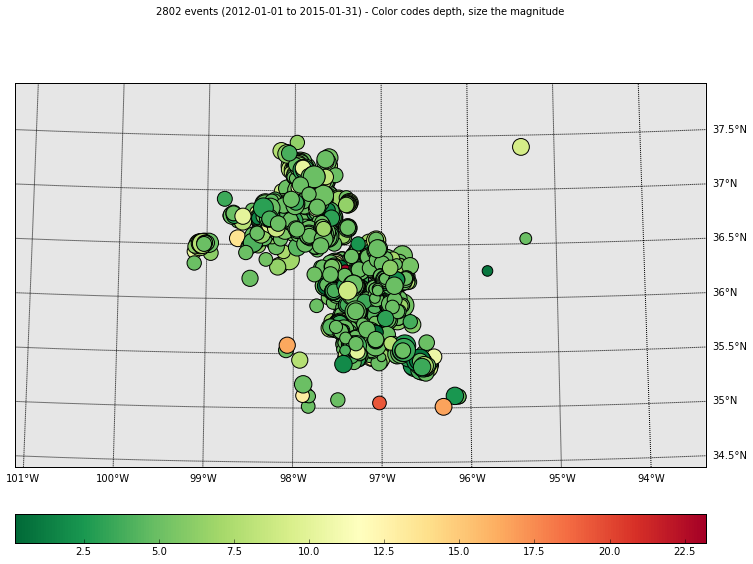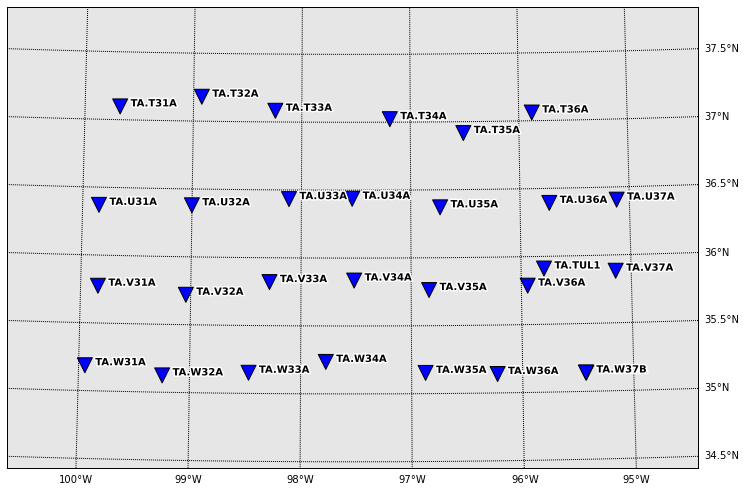Unfortunately, we cannot use ObsPy directly to plot both events and stations on the same plot. We must instead use Python and matplotlib's map plotting functionality directly. Fortunately, it is not too difficult to achieve.

Simply create a plot with the desired projection, and draw a scatter of the points as done usually.

In :
from mpl_toolkits.basemap import Basemap
from obspy.core.util import degrees2kilometers

ev_lat = [ev.preferred_origin().latitude for ev in ok_ev]
ev_lon = [ev.preferred_origin().longitude for ev in ok_ev]
sta_lat = [sta.latitude for net in ok_sta for sta in net]
sta_lon = [sta.longitude for net in ok_sta for sta in net]

lon = np.array(ev_lon + sta_lon)
lat = np.array(ev_lat + sta_lat)
avg_lon = np.mean(lon)
avg_lat = np.mean(lat)
width = 1.1e3 * degrees2kilometers(np.max(lon) - np.min(lon))
height = 1.1e3 * degrees2kilometers(np.max(lat) - np.min(lat))

bm = Basemap(projection='aea',
lon_0=avg_lon, lat_0=avg_lat, width=width, height=height)
bm.drawstates()
parallels = np.arange(int(bm.llcrnrlat), int(bm.urcrnrlat) + 1, 0.5)
bm.drawparallels(parallels, labels=[1,0,0,0], fontsize=10, dashes=[1, 7])
meridians = np.arange(int(bm.llcrnrlon) - 1, int(bm.urcrnrlon), 0.5)
bm.drawmeridians(meridians,labels=[0,0,0,1],fontsize=10, dashes=[1, 7])

bm.scatter(np.array(ev_lon), np.array(ev_lat), latlon=True,
s=100, c='r', alpha=0.3, edgecolor='none', marker='.',
label='Earthquake')
bm.scatter(np.array(sta_lon), np.array(sta_lat), latlon=True,
s=100, c='b', edgecolor='none', marker='v',
label='TA station')

plt.legend(loc='upper left', scatterpoints=1)
plt.title(title)
Out:
<matplotlib.text.Text at 0x7f8166fdaba8>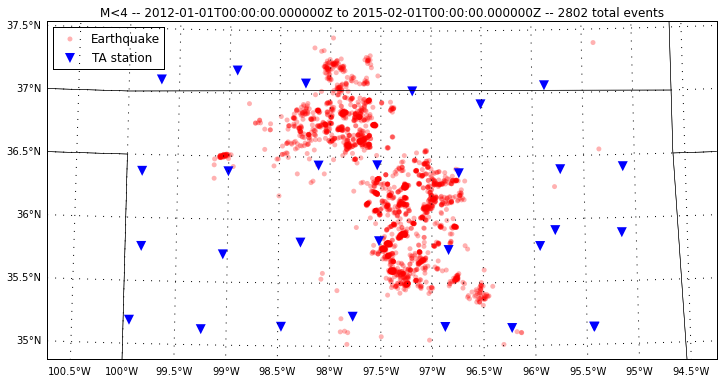Example 3¶

Retrieving data from other datacenters. The versatility of the obspy.fdsn methods allow users to access data from web services hosted by other datacenters, as long as they conform to the specification set by the FDSN. This example uses data obtained from the USGS fdsn-event service.

In :
usgs = Client('USGS')

minmag_glob = 6
tstart = UTCDateTime('2000-01-01T00:00:00')
glob_ev = usgs.get_events(starttime=tstart, minmagnitude=minmag_glob)
In :
fig = glob_ev.plot(color='date')